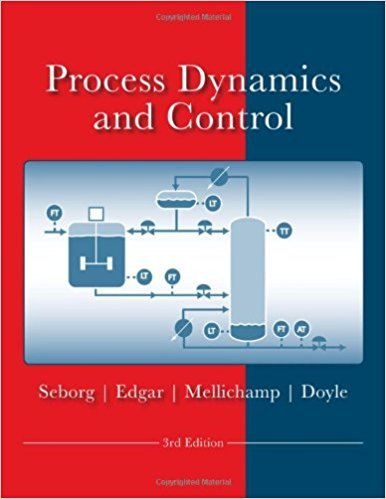×
Get Full Access to Process Dynamics And Control - 3 Edition - Chapter 14 - Problem 14.1
Get Full Access to Process Dynamics And Control - 3 Edition - Chapter 14 - Problem 14.1

×

# A heat transfer process has the following transferISBN: 9780470128671 148

## Solution for problem 14.1 Chapter 14

Process Dynamics and Control | 3rd Edition

• Textbook Solutions
• 2901 Step-by-step solutions solved by professors and subject experts
• Get 24/7 help from StudySoup virtual teaching assistantsProcess Dynamics and Control | 3rd Edition

4 5 1 405 Reviews
28
3
Problem 14.1

A heat transfer process has the following transfer function between a temperature T and an inlet flow rate q where the time constants have units of minutes: T'(s) 3(1-s) Q'(s) s(2s+1) If the flow rate varies sinusoidally with an amplitude of 2 Llmin and a period of 0.5 min, what is the amplitude of the temperature signal after the transients have died out?

Step-by-Step Solution:
Step 1 of 3

Step 2 of 3

Step 3 of 3

##### ISBN: 9780470128671

The answer to “A heat transfer process has the following transfer function between a temperature T and an inlet flow rate q where the time constants have units of minutes: T'(s) 3(1-s) Q'(s) s(2s+1) If the flow rate varies sinusoidally with an amplitude of 2 Llmin and a period of 0.5 min, what is the amplitude of the temperature signal after the transients have died out?” is broken down into a number of easy to follow steps, and 63 words. The full step-by-step solution to problem: 14.1 from chapter: 14 was answered by , our top Engineering and Tech solution expert on 11/15/17, 04:03PM. This full solution covers the following key subjects: amplitude, transfer, temperature, flow, rate. This expansive textbook survival guide covers 24 chapters, and 420 solutions. This textbook survival guide was created for the textbook: Process Dynamics and Control, edition: 3. Since the solution to 14.1 from 14 chapter was answered, more than 592 students have viewed the full step-by-step answer. Process Dynamics and Control was written by and is associated to the ISBN: 9780470128671.

Unlock Textbook Solution